Function Repository Resource:

# SimpleCausalGraphCoordinates

Generate Cartesian-like coordinates for a simple two-dimensional causal graph

Contributed by: Tom Lee, Jon Lederman
 ResourceFunction["SimpleCausalGraphCoordinates"][size,type] returns coordinates of the form {pointnumber,time,space} for the causal graph of structural type type.

## Details

size represents the initial node size from which the resulting causal graph is built; the first column of the result will contain size coordinates.
Supported values for type are "Triangular", "Hexagonal" and "TriangularHexagonal". These describe the lattice on which the points lie in 1+1 dimensional spacetime.

## Examples

### Basic Examples (3)

Coordinates for the simple triangular type causal graph with initial node size 10:

 In:=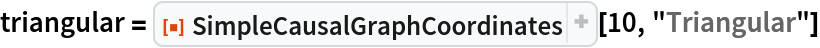Out=Check that lie on a triangular lattice:

 In:=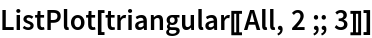Out=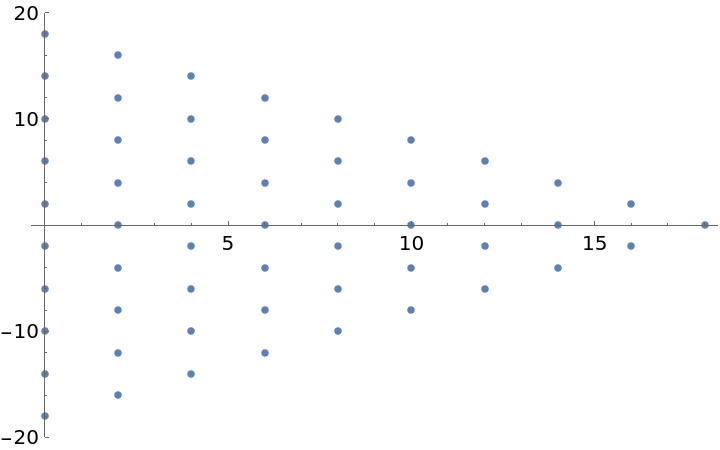Coordinates for the hexagonal type causal graph with initial node size 10:

 In:=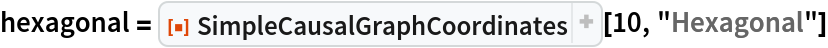Out=Check that these lie on a hexagonal lattice:

 In:=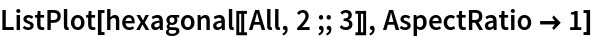Out=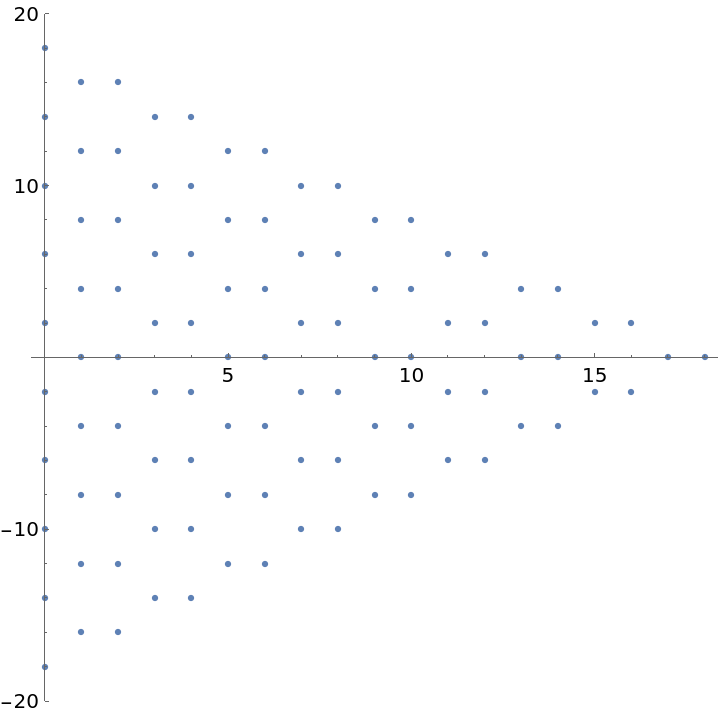Coordinates for the hybrid triangular-hexagonal type causal graph with initial node size 10:

 In:=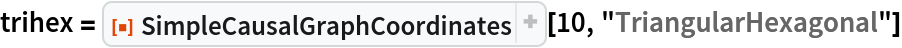Out=Check that these lie on a hybrid lattice with triangles between hexagons:

 In:=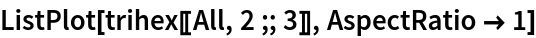Out=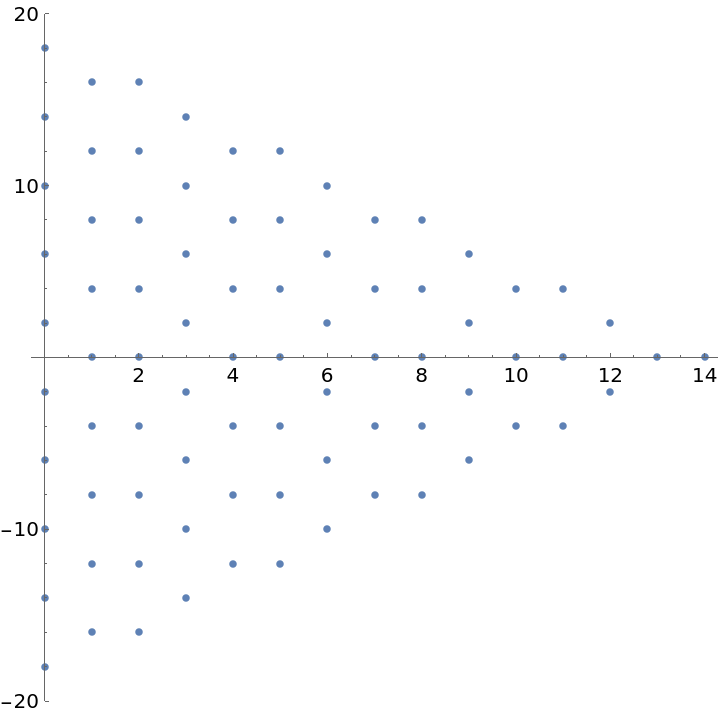### Scope (2)

Create a triangular structured causal graph:

 In:=Out=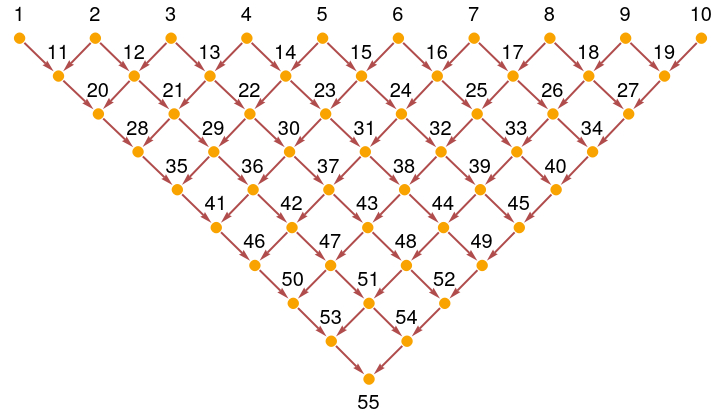Calculate the coordinates for each node:

 In:=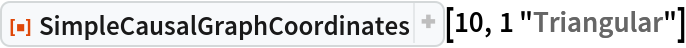Out=Tom Lee

## Version History

• 3.0.0 – 11 June 2022
• 2.0.0 – 14 March 2022
• 1.0.0 – 04 October 2021